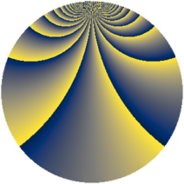# Properties

 Label 1694.2.beLevel $1694$ Weight $2$ Character orbit 1694.be Rep. character $\chi_{1694}(17,\cdot)$ Character field $\Q(\zeta_{330})$ Dimension $7040$ Sturm bound $528$

# Related objects

## Defining parameters

 Level: $$N$$ $$=$$ $$1694 = 2 \cdot 7 \cdot 11^{2}$$ Weight: $$k$$ $$=$$ $$2$$ Character orbit: $$[\chi]$$ $$=$$ 1694.be (of order $$330$$ and degree $$80$$) Character conductor: $$\operatorname{cond}(\chi)$$ $$=$$ $$847$$ Character field: $$\Q(\zeta_{330})$$ Sturm bound: $$528$$

## Dimensions

The following table gives the dimensions of various subspaces of $$M_{2}(1694, [\chi])$$.

Total New Old
Modular forms 21440 7040 14400
Cusp forms 20800 7040 13760
Eisenstein series 640 0 640

## Trace form

 $$7040q + 88q^{4} - 12q^{5} + 10q^{7} - 892q^{9} + O(q^{10})$$ $$7040q + 88q^{4} - 12q^{5} + 10q^{7} - 892q^{9} + 66q^{10} - 14q^{11} + 24q^{14} + 100q^{15} - 88q^{16} + 30q^{17} + 80q^{22} + 16q^{23} + 100q^{25} + 24q^{26} - 10q^{28} - 20q^{29} + 18q^{31} + 126q^{33} - 30q^{35} + 192q^{36} + 46q^{37} + 12q^{38} - 20q^{39} + 30q^{40} + 10q^{42} + 2q^{44} - 108q^{45} + 12q^{47} - 102q^{49} + 16q^{51} + 18q^{53} - 4q^{56} + 80q^{57} + 24q^{58} + 60q^{59} - 4q^{60} - 30q^{61} - 160q^{63} - 176q^{64} - 72q^{66} - 72q^{67} - 30q^{68} + 2q^{70} + 8q^{71} - 20q^{72} - 90q^{73} - 20q^{74} - 204q^{75} - 134q^{77} - 32q^{78} - 118q^{79} - 18q^{80} + 832q^{81} - 36q^{82} - 40q^{84} + 300q^{85} - 18q^{86} - 14q^{88} - 378q^{89} - 148q^{91} - 204q^{92} - 136q^{93} + 120q^{94} - 106q^{95} - 88q^{98} - 324q^{99} + O(q^{100})$$

## Decomposition of $$S_{2}^{\mathrm{new}}(1694, [\chi])$$ into newform subspaces

The newforms in this space have not yet been added to the LMFDB.

## Decomposition of $$S_{2}^{\mathrm{old}}(1694, [\chi])$$ into lower level spaces

$$S_{2}^{\mathrm{old}}(1694, [\chi]) \cong$$ $$S_{2}^{\mathrm{new}}(847, [\chi])$$$$^{\oplus 2}$$